# Resonant Converter

Description of the Resonant Converter component in Schematic Editor. This converter block uses the dedicated DC-DC Converter solver FPGA hardware resource in order to achieve a reduced simulation step for this topology.

The Resonant Converter component supports two main topologies: CLLLC Resonant Converter and LLC Resonant Converter.

## CLLLC Resonant Converter

A block diagram and input parameters for the CLLLC Resonant Converter are given in Table 1.

Table 1. CLLLC resonant converter component in Schematic Editor
component component dialog window component properties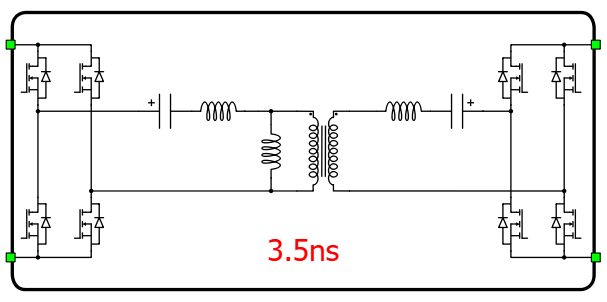Resonant Converter• Control
• Control (Digital inputs, Internal modulator)
• SA_1 (digital input selection for switch SA_1)
• SA_2 (digital input selection for switch SA_2)
• SA_3 (digital input selection for switch SA_3)
• SA_4 (digital input selection for switch SA_4)
• SB_1 (digital input selection for switch SB_1)
• SB_2 (digital input selection for switch SB_2)
• SB_3 (digital input selection for switch SB_3)
• SB_4 (digital input selection for switch SB_4)
• gate_logic (active high, active low)
• Gate control enabling - A (enables usage of an external digital signal to enable the switching of side A)
• Sen - A (digital input selection for PWM enable for side A)
• Sen_logic - A (active high, active low)
• Gate control enabling - B (enables usage of an external digital signal to enable the switching of side B)
• Sen - B (digital input selection for PWM enable for side B)
• Sen_logic - B (active high, active low)
• Electrical
• Resonant tank (Type of Resonant Converter tank - LLC or CLLLC)
• A side topology (Converter topology at A side - if CLLLC is selected, only H-bridge is available)
• B side topology (Converter topology at B side - if CLLLC is selected, only H-bridge is available)
• Series inductance (A) - (Series Resonant inductance at A side)
• Capacitance (A) - (Series Resonant capacitance at A side)
• Parallel inductance (Inductance of parallel inductor)
• Series inductance (B) - (Series Resonant inductance at B side)
• Capacitance (B) - (Series Resonant capacitance at B side)
• Transformer turns ratio (B/A) (Defines internal transformer ratio between sides B and A)
• Extras
• Short-circuit resistance - A (Resistance for side A used to calculate short circuit current if side A is shorted)
• Short-circuit resistance - B (Resistance for side B used to calculate short circuit current if side B is shorted)

Digital inputs, when selected as the Control parameter, enables you to assign gate drive inputs to any of the digital input pins. For example, if SA_1 is assigned to 1, the digital input pin 1 will be routed to the SA_1 switch gate drive. In addition, the SA1_logic parameter is set to either active high (i.e. high-level input voltage VIH which turns on the switch), or active low (i.e. low-level input voltage VIL which turns on the switch). The gate drive logic depends on your external controller design.

Internal modulator, when selected for Control parameter, enables you to use the internal PWM modulator for driving switches instead of digital input pins. In this configuration, additional component inputs will be present. En_A and En_B input is used to enable/disable switching at corresponding side. In_a1, In_a2, In_b1, and In_b2 are used as referent signal inputs for the corresponding internal PWM modulator in legs A1, A2, B1, and B2, respectively. Additionally, Offset_a1, Offset_a2, Offset_b1, and Offset_b2 are used as carrier offsets for the corresponding internal PWM modulator in legs A1, A2, B1, and B2, respectively. If Variable carrier frequency is selected as the Operation mode for the CLLLC Resonant Converter's internal modulator, an additional port Freq will be present. This port is used as a frequency input for the internal PWM modulator.

Short-circuit resistance - A and Short-circuit resistance - B are used to define the resistance that will be taken into consideration if some of the legs enter short-circuit mode. Short-circuits are modelled using short-circuit resistance, which means that if a leg is in short-circuit mode, current drawn from its corresponding DC side will be Vdc_A(B)/Short-circuit resistance - A(B), where Vdc_A(B) is the DC voltage of the corresponding DC side.

In Figure 1 you can find the placement of all electrical components, as well as names of all converter switches.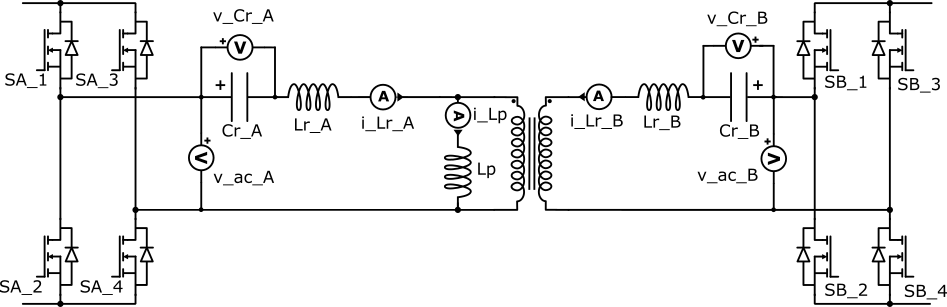Figure 1. A schematic block diagram of a CLLLC Resonant Converter component with corresponding switch naming

## LLC Resonant Converter

A block diagram and input parameters for the LLC Resonant Converter are given in Table 2.

Table 2. LLC resonant converter component in Schematic Editor
component component dialog window component propertiesResonant Converter• Control
• Control (Digital inputs, Internal modulator)
• SA_1 (digital input selection for switch SA_1)
• SA_2 (digital input selection for switch SA_2)
• SA_3 (digital input selection for switch SA_3 - availiable if A side topology is H-bridge)
• SA_4 (digital input selection for switch SA_4 availiable if A side topology is H-bridge)
• SB_1 (digital input selection for switch SB_1 - availiable if B side topology contains controllable switch SB_1)
• SB_2 (digital input selection for switch SB_2 - availiable if B side topology contains controllable switch SB_2)
• SB_3 (digital input selection for switch SB_3 - availiable if B side topology contains controllable switch SB_3)
• SB_4 (digital input selection for switch SB_4 - availiable if B side topology contains controllable switch SB_4)
• gate_logic (active high, active low)
• Gate control enabling - A (enables usage of an external digital signal to enable the switching of side A)
• Sen - A (digital input selection for PWM enable for side A)
• Sen_logic - A (active high, active low)
• Gate control enabling - B (enables usage of an external digital signal to enable the switching of side B)
• Sen - B (digital input selection for PWM enable for side B)
• Sen_logic - B (active high, active low)
• Electrical
• Resonant tank (Type of Resonant Converter tank - LLC or CLLLC)
• A side topology (Converter topology at A side - H-bridge, Half-bridge, Half-bridge split-cap)
• B side topology (Converter topology at B side - H-bridge, Full bridge rectifier, Active Center-tapped, Diode Center-tapped)
• Series inductance (A) - (Series Resonant inductance at A side)
• Capacitance (A) - (Series Resonant capacitance at A side. If the Half bridge split-cap topology is selected, this property represents total capacitance of both capacitors at the A side.)
• Parallel inductance (Inductance of parallel inductor)
• Transformer turns ratio (B/A) (Defines internal transformer ratio between sides B and A. If the Center-tapped topology is selected, all secondary windings are considered as same and B represents number of turns of single winding.)
• Extras
• Short-circuit resistance - A (Resistance for side A used to calculate short circuit current if side A is shorted)
• Short-circuit resistance - B (Resistance for side B used to calculate short circuit current if side B is shorted)

If LLC is selected as the Resonant tank, different A and B side topologies can be selected. Table 3 gives an overview of A side topologies, and Table 4 gives an overview of B side topologies.

Table 3. A side topologies
Name Topology

H-bridge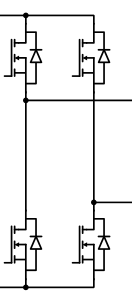Half bridge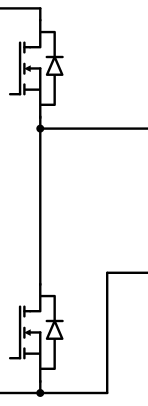Half bridge split-caps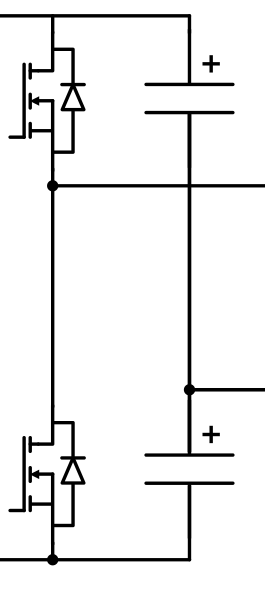Table 4. B side topologies
Name Topology

H-bridge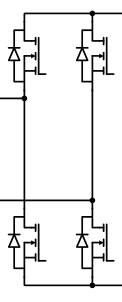Full bridge rectifier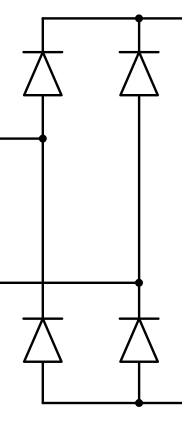Active Center-tapped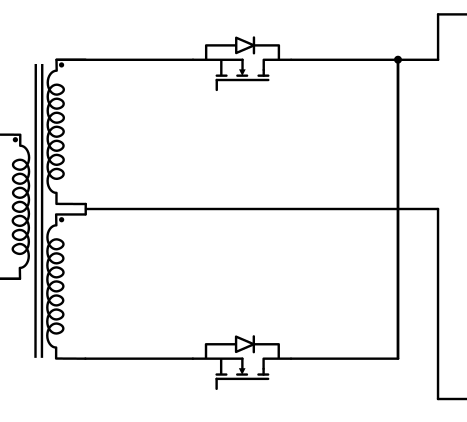Diode Center-tapped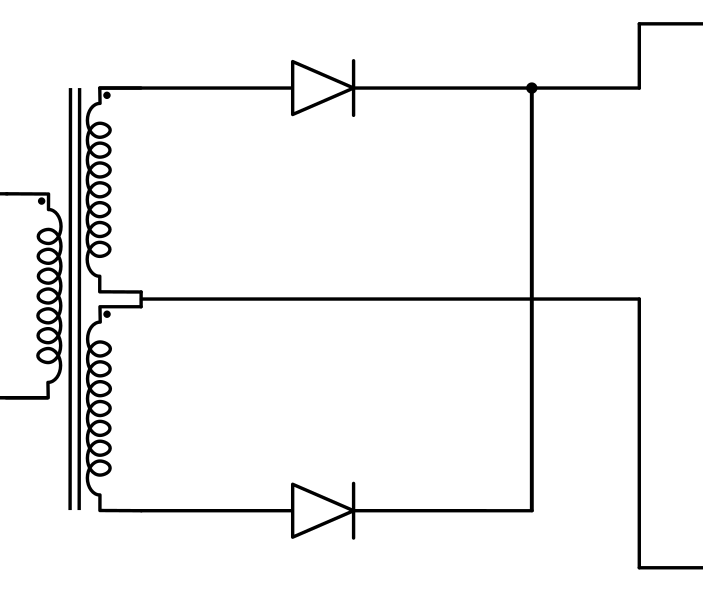Digital inputs, when selected as the Control parameter, enables you to assign gate drive inputs to any of the digital input pins. For example, if SA_1 is assigned to 1, the digital input pin 1 will be routed to the SA_1 switch gate drive. In addition, the SA1_logic parameter is set to either active high (i.e. high-level input voltage VIH which turns on the switch), or active low (i.e. low-level input voltage VIL which turns on the switch). The gate drive logic depends on your external controller design.

Internal modulator, when selected for Control parameter, enables you to use the internal PWM modulator for driving switches instead of digital input pins. Internal modulator drives only switches at A side, while controllable switches at B side (if any exists in selected B topology) will be turned off, so the B side will behave as completely passive. In this configuration, additional component inputs will be present. En input is used to enable/disable switching. In_a1 and In_a2 are used as referent signal inputs for the corresponding internal PWM modulator in legs A1 and A2. Additionally, Offset_a1, Offset_a2 are used as carrier offsets for the corresponding internal PWM modulator in legs A1 and A2, respectively. If the A side topology is not H-bridge, In_a2 and Offset_a2 will not exist. If Variable carrier frequency is selected as the Operation mode for the LLC Resonant Converter's internal modulator, an additional port Freq will be present. This port is used as a frequency input for the internal PWM modulator.

Short-circuit resistance - A and Short-circuit resistance - B are used to define the resistance that will be taken into consideration if some of the legs enter short-circuit mode. Short-circuits are modelled using short-circuit resistance, which means that if a leg is in short-circuit mode, current drawn from its corresponding DC side will be Vdc_A(B)/Short-circuit resistance - A(B), where Vdc_A(B) is the DC voltage of the corresponding DC side.

In Figure 1 you can find the placement of all electrical components, as well as names of all converter switches if both the A side topology and the B side topology are H-bridge. For other combinations of A side topology and B side topology, refer to the block diagrams at the end of the document.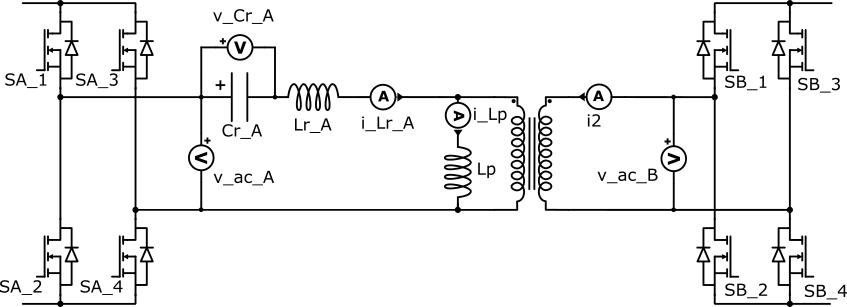Figure 2. A schematic block diagram of a LLC Resonant Converter (H-bridge, H-bridge) component with corresponding switch naming

## Model description

The Resonant Converter runs on the DC-DC Converter Solver dedicated hardware solver module, which is highly optimized to simulate the dynamics of fast-switching converter topologies with enhanced resolution. This means that the Resonant Converter block does not utilize the same resources that are typically used for other converter blocks, so the weight of the Resonant Converter is 0.

Note: Virtual HIL (VHIL) currently does not support this converter.

## Electrical circuit interface

As described in Electrical circuit interface, every component that uses the DC-DC Converter Solver hardware resource contains an interfacing electrical circuit towards the rest of the circuit. The Resonant Converter component in the Typhoon HIL Schematic Editor Library uses the current source interface. The interface is formulated in such a way that the voltages are inputs to the dedicated DC-DC Converter solver core, while the currents are outputs from the dedicated DC-DC Converter solver core. Figure 3 shows the circuit interface of the Resonant Converter comoponent. Besides IA and IB which directly affect the surrounding circuit, there are additional component outputs that represent internal electrical measurements. Table 5 shows measurements available depending on the topology.
Table 5. Feedback measurements
Resonant tank A side topology B side topology Measurements

CLLLC

H-bridge

H-bridge

i_Lr_A, i_Lr_B, i_Lp, v_Cr_A, v_Cr_B, v_ac_A, v_ac_B

LLC

H-bridge, Half bridge

all

i_Lr_A, i2, i_Lp, v_Cr_A, v_ac_A, v_ac_B

LLC

Half bridge split-cap

all

i_Lr_A, i2, i_Lp, v_Cr_A_Top, v_Cr_A_Bot, v_ac_A, v_ac_B

Placement of all measurements for all topologies is shown in the Block diagram(s) of a Resonant Converter component with corresponding switch naming section.